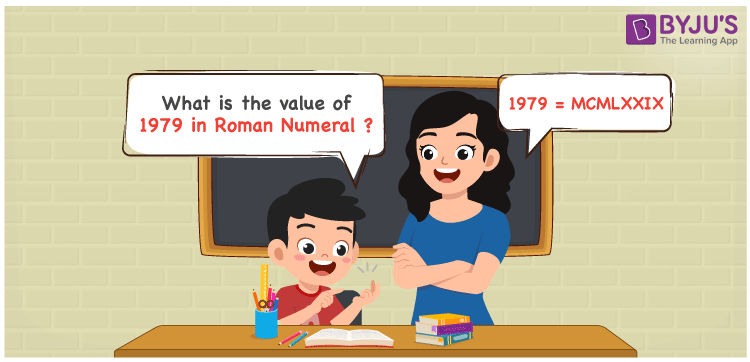# 1979 in Roman Numerals

1979 in Roman Numerals is MCMLXXIX. Any Roman numeral uses only the 7 primary symbols which are I, V, X, L, C, D, and M, Where M represents 1000, C represents 100, L represents 50, X represents 10 and I represents 1. MCMLXXIX represents the number 1979 which is obtained by the addition and subtraction rules of Roman Numerals. What are Roman numerals? can be referred for more details.

 Number Roman Numeral 1979 MCMLXXIX

## How to Write 1979 in Roman Numerals?To convert 1979 in Roman Numerals, we need to represent 1979 as the sum of the fundamental symbols. 1979 can be broken down as

1. The number 1000,
2. Difference of 1000 and 100 to get 900
3. Add 50 to twice of 10 to get 70
4. Difference of 10 and 1 to get 9.
5. Add all the numbers from previous steps.

Let us understand it with Numerical representation.

1979 = 1000 + 900 + 70 + 9

1979 = 1000 + (1000 – 100) + (50 + 10 + 10 ) + ( 10 – 1)

1979 = M + (M – C) + (L + X + X) + (X – I)

1979 = M + (CM) + LXX + (IX)

1979 = MCMLXXIX

## Video Lesson on Roman Numerals## Frequently Asked Questions on 1979 in Roman Numerals

### How to write the number 1979 in Roman Numerals?

1979 in Roman Numerals is written as MCMLXXIX.

### Does MCMLXXIX represent Roman Numerals?

Yes. MCMLXXIX represents Roman Numerals and it is the number 1979

### What is the year 1979 in Roman numerals?

The year 1979 in Roman Numerals is MCMLXXIX.